##### PostgreSQL Numeric Data Type With Practical Examples

Numeric data types are an essential part of any database system, and PostgreSQL is no exception. These data types allow us to store and manipulate numbers in various formats, from simple integers to complex decimal values. These data types include INTEGER, NUMERIC, BIGINT, etc., each of which is designed to store different types of numerical values.

The NUMERIC data type is a more precise data type that is used to store decimal values. It can be defined with specific precision and scale, allowing us to specify the number of decimal places to be stored.

This blog will explain the usage of the NUMERIC data type in Postgres via Practical examples. So, let’s start!

PostgreSQL Numeric Data Type

The NUMERIC data type is a high-precision decimal data type in Postgres that is suitable for storing numbers requiring many decimal places. Here is the syntax to use the NUMERIC data type in Postgres:

`NUMERIC(precision, scale);`

Here, the precision represents the total number of digits, while the scale parameter represents the number of fractional digits. For instance, a numeric value “13411.267” has a precision “8” and a scale “3”. The scale parameter is optional and can be omitted. Omitting the scale part will store the numeric values without the fractional part.

Example 1: Create a Column With NUMERIC Data Type (With Scale)

In this example, we will create a table named “emp_data” with three columns: “emp_id”, “emp_name”, and “emp_salary”:

```CREATE TABLE emp_data (
emp_id SERIAL PRIMARY KEY,
emp_name TEXT NOT NULL,
emp_salary NUMERIC(6,2)
);```

In this example, we created a column with NUMERIC data type having precision “6” and scale “2”: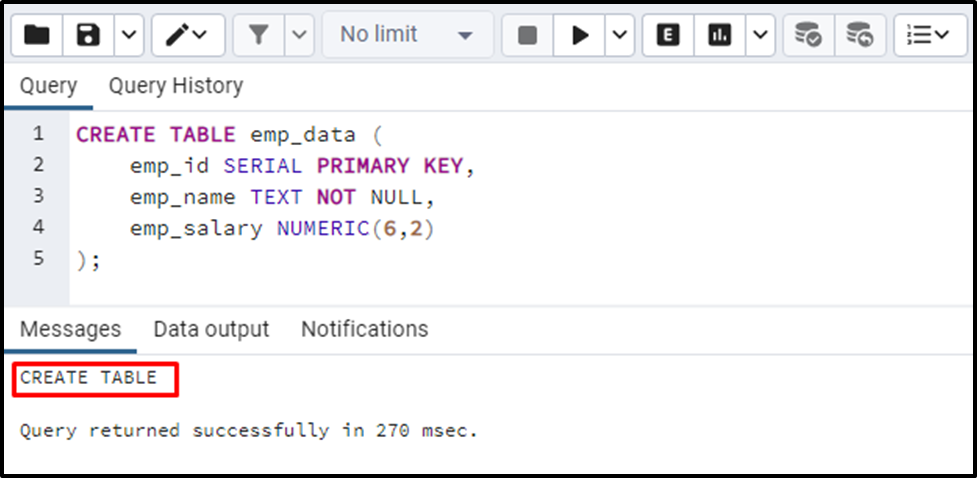The above snippet verifies that a table named emp_data with three columns has been created successfully. To insert the data into the “emp_data” table, you need to run the “INSERT INTO” statement as follows:

```INSERT INTO emp_data (emp_name, emp_salary)
VALUES('Tim', 5500.5555),
('David', 4500.1234),
('Joseph', 4000.412),
('Joe', 3500.515),
('John', 3850.7123);```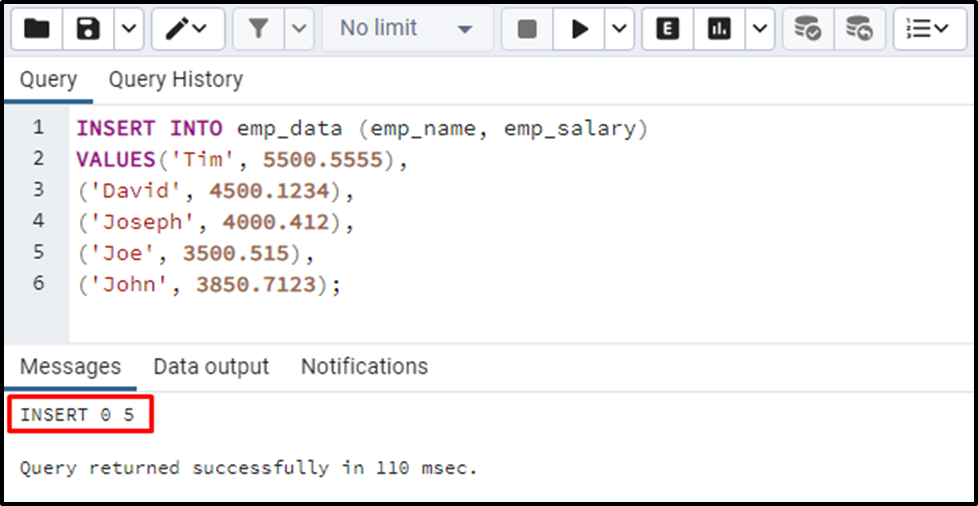Let’s query the table’s data via the below-provided command:

`SELECT * FROM emp_data;`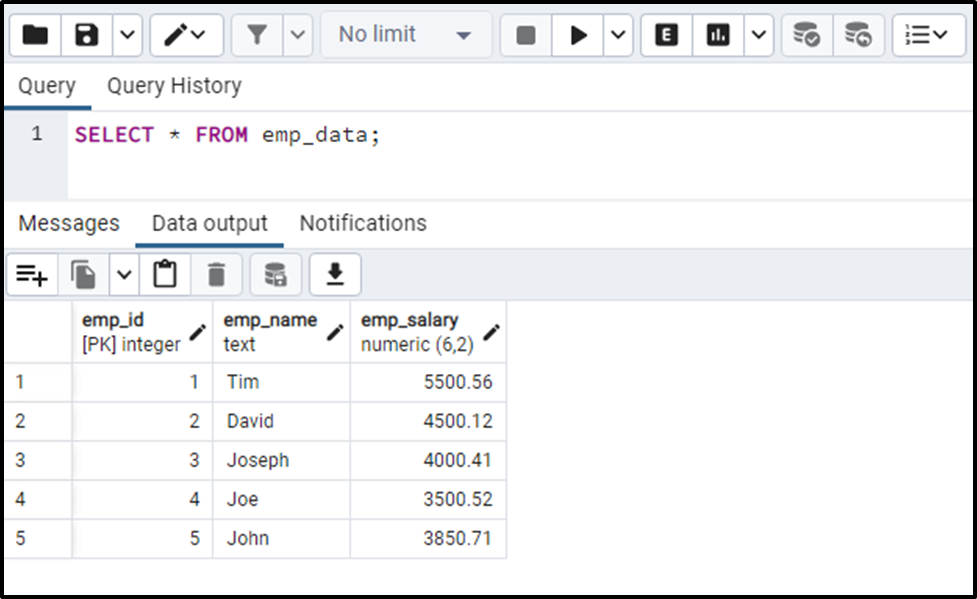From the output, you can observe that the fractional part has been trimmed to two decimal places because the scale was set to “2”.

Example 2: Create a Column With NUMERIC Data Type (Without Scale)

In this example, we will show you the usage of NUMERIC data type without the “scale” parameter:

```CREATE TABLE emp_information (
emp_id SERIAL PRIMARY KEY,
emp_name TEXT NOT NULL,
emp_salary NUMERIC(6)
);```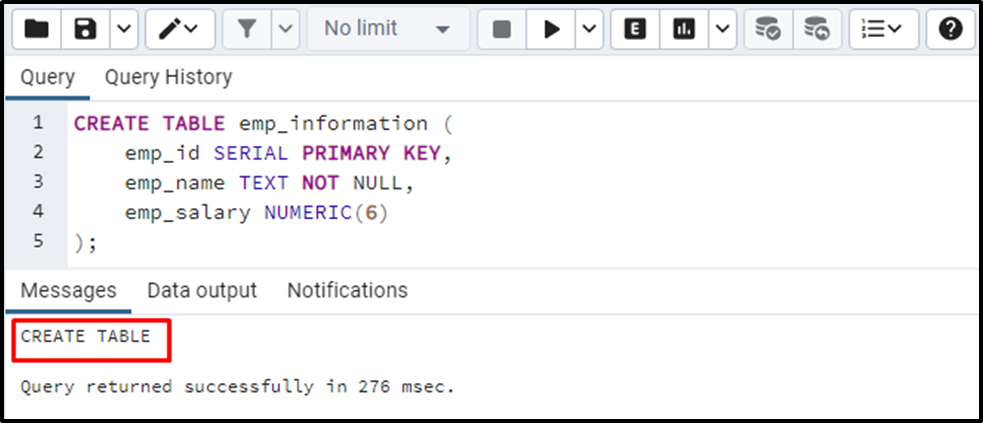The table with desired columns has been created. Now, it's time to insert the employee information into the newly created “employee_information” table:

```INSERT INTO emp_information (emp_name, emp_salary)
VALUES('Tim', 5500.5555),
('David', 4500.1234),
('Joseph', 4000.412),
('Joe', 3500.515),
('John', 3850.7123);```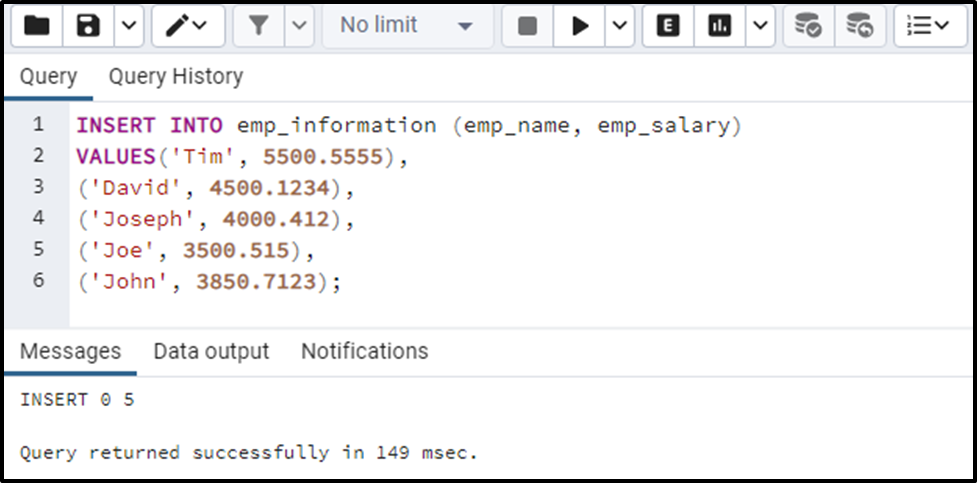Now execute the “SELECT *” command to verify the data insertion:

`SELECT * FROM emp_information;`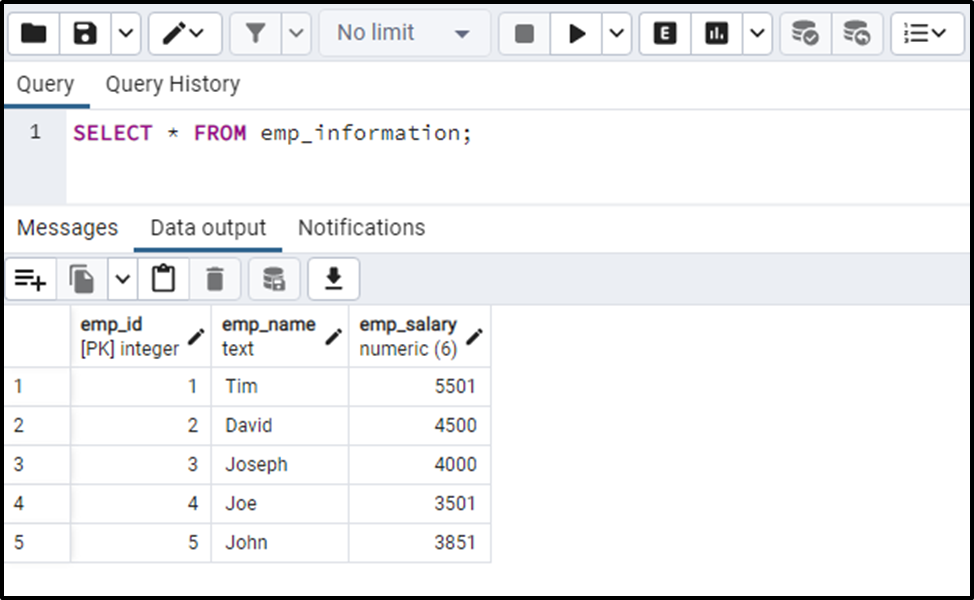The output snippet shows that the NUMERIC values are stored in the table without fractional parts. This is how the NUMERIC data type works if you omit the “scale” parameter.

That’s all from this Postgres guide!

Conclusion

The NUMERIC data type is a more precise data type used to store decimal values. It can be defined with specific precision and scale, allowing us to specify the number of decimal places to be stored. The scale parameter is optional and can be omitted. Omitting the scale part will store the numeric values without the fractional part. This blog post explained what NUMERIC data type is, its basic syntax, and suitable examples.